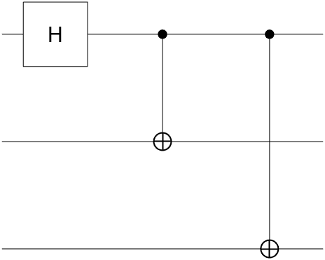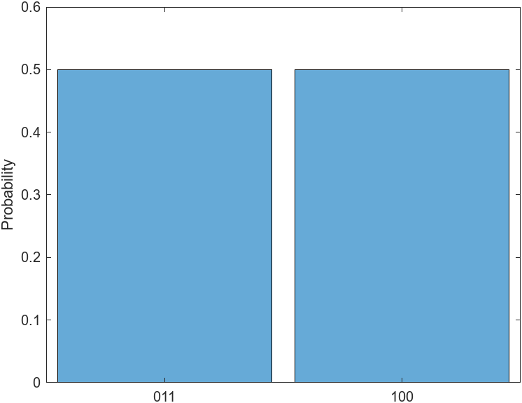## Local Quantum State Simulation

Note

Installation Required: This functionality requires MATLAB® Support Package for Quantum Computing. Install the support package from Add-On Explorer using the instructions in Get and Manage Add-Ons. Alternatively, see MATLAB Support Package for Quantum Computing on File Exchange.

This topic describes how to simulate a quantum circuit locally and analyze the simulation results. Building quantum circuits requires iteration on circuit design to refine circuit gates and confirm behavior. Also, because measurements on quantum hardware are probabilistic, it is useful to examine the probabilities of final states to determine the most likely outcome of a measurement. Use the `simulate` method of `quantumCircuit` to simulate circuits on your local computer. Once you simulate a circuit, use the methods of `quantum.gate.QuantumState` to inspect the results.

### Create Circuit

Create a quantum circuit with three qubits and three gates. Apply a Hadamard gate to the first qubit, and apply CNOT gates to the second and third qubits, using the first qubit as the control. Plot the circuit to view its qubits and gates.

```gates = [hGate(1); cxGate(1,2); cxGate(1,3)]; C = quantumCircuit(gates); plot(C)```Each horizontal line in the plot represents one of the qubits, and the gates are arranged from left to right in the order that they are applied.

### Simulate Circuit

Simulate the quantum circuit by using the `simulate` method. All qubits start in the $|0〉$ state by default, but you can use a second input argument to specify a different starting state for the qubits. Specify that each qubit has an initial state of $|1〉$.

`S = simulate(C,"111")`
```S = QuantumState with properties: BasisStates: [8×1 string] Amplitudes: [8×1 double] NumQubits: 3```

Display the basis states and corresponding amplitudes by inspecting the properties of the resulting `quantum.gate.QuantumState` object.

`S.BasisStates`
```ans = 8×1 string array "000" "001" "010" "011" "100" "101" "110" "111"```
`S.Amplitudes`
```ans = 0 0 0 0.7071 -0.7071 0 0 0```

### Display State Formula

Display a formula representation of the simulated quantum state by using the `formula` method. The formula combines the information in the `BasisStates` and `Amplitudes` properties of `S`. By default, the formula uses the Z basis.

`f = formula(S)`
```f = "0.70711 * |011> + -0.70711 * |100>"```

Specify the `Basis` name-value argument to display the formula using the X basis. The formula now shows linear combinations of $|+〉$ and $|-〉$ states.

`f2 = formula(S,Basis="X")`
```f2 = "-0.5 * |++-> + -0.5 * |+-+> + 0.5 * |-++> + 0.5 * |--->"```

### Plot Possible States

Use `histogram` to plot the possible states and their probabilities. The probability of each state is equal to its amplitude squared. This circuit has only two possible states, and each state has a 50% chance of being measured.

`histogram(S)`### Query Possible States

You can query the possible states and their probabilities using the `querystates` method. This method returns the same information that the histogram shows, but at the command line.

`[states,P] = querystates(S)`
```states = 2×1 string array "011" "100" P = 0.5000 0.5000```

### Query Qubit State Probabilities

You can query the probability that specific qubits will be measured in specific states by using the `probability` method. For example, determine the probability that the second qubit will be measured in the $|1〉$ state.

`p = probability(S,2,"1")`
```p = 0.5000```

### Simulate Quantum State Measurement

Quantum measurements are probabilistic, so the results can differ between trials. The `randsample` method is useful for simulating the aggregated results of many such measurements. You can specify the number of shots, or trials, and each shot returns a single state.

For example, simulate 50 quantum measurements of the circuit.

`M = randsample(S,50)`
```M = QuantumMeasurement with properties: MeasuredStates: [2×1 string] Counts: [2×1 double] NumQubits: 3```

Display the measured states and their counts.

`T = table(M.Counts,M.MeasuredStates,VariableNames=["Counts","States"])`
```T = 2×2 table Counts States ______ ______ 21 "011" 29 "100" ```